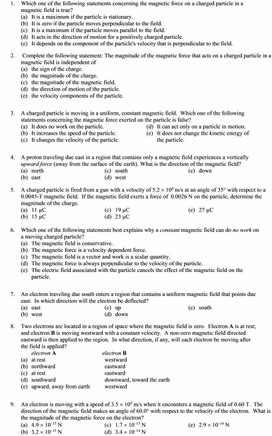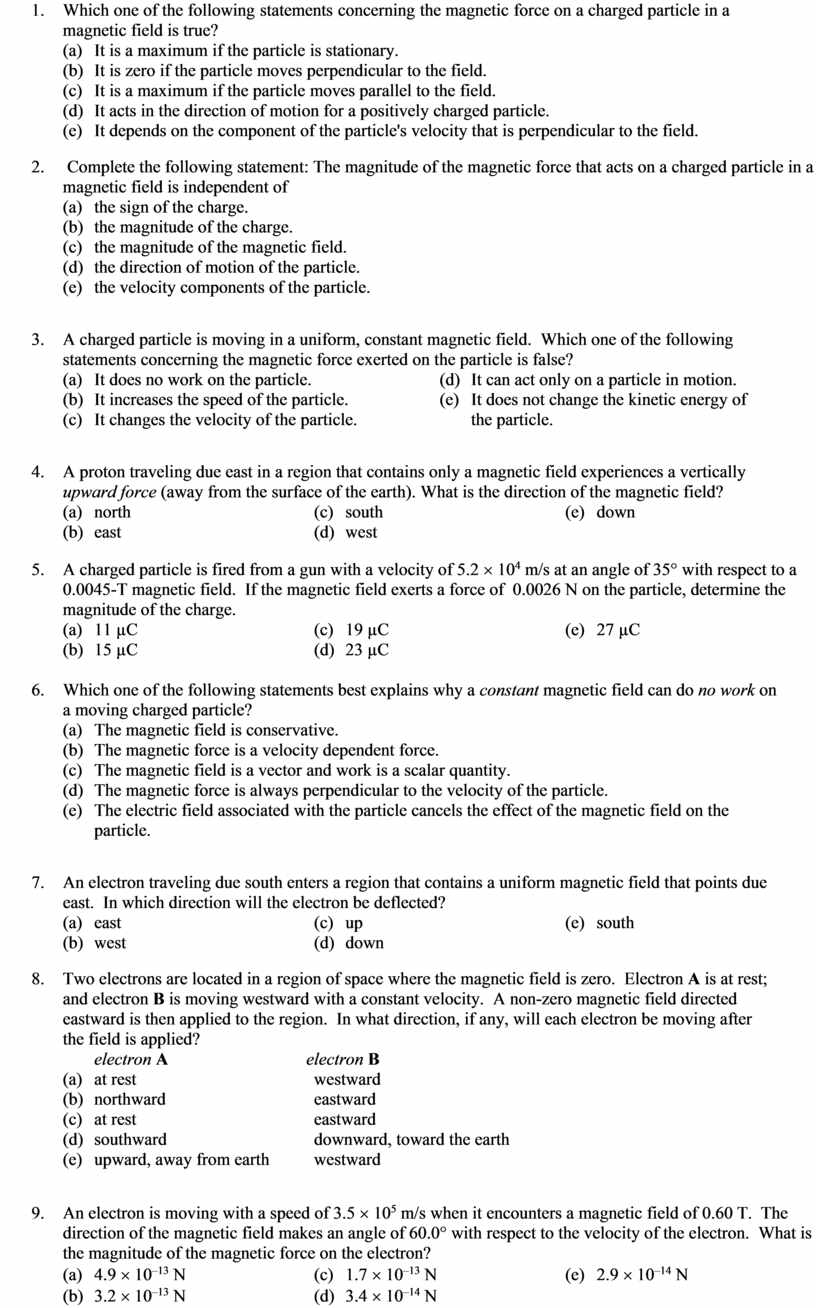# Сұрақ-жауап

Оценка 4.7
docx
07.05.2020сұрақ-жауап.docx

1.       Which one of the following statements concerning the magnetic force on a charged particle in a magnetic field is true?

(a)   It is a maximum if the particle is stationary.

(b)   It is zero if the particle moves perpendicular to the field.

(c)   It is a maximum if the particle moves parallel to the field.

(d)   It acts in the direction of motion for a positively charged particle.

(e)   It depends on the component of the particle's velocity that is perpendicular to the field.

2.        Complete the following statement: The magnitude of the magnetic force that acts on a charged particle in a magnetic field is independent of

(a)   the sign of the charge.

(b)   the magnitude of the charge.

(c)   the magnitude of the magnetic field.

(d)   the direction of motion of the particle.

(e)   the velocity components of the particle.

3.       A charged particle is moving in a uniform, constant magnetic field.  Which one of the following statements concerning the magnetic force exerted on the particle is false?

(a)   It does no work on the particle.                                 (d)   It can act only on a particle in motion.

(b)   It increases the speed of the particle.                       (e)   It does not change the kinetic energy of

(c)   It changes the velocity of the particle.                             the particle.

4.       A proton traveling due east in a region that contains only a magnetic field experiences a vertically upward force (away from the surface of the earth). What is the direction of the magnetic field?

(a)   north                                              (c)   south                                              (e)   down

(b)   east                                                 (d)   west

5.       A charged particle is fired from a gun with a velocity of 5.2 ´ 104 m/s at an angle of 35° with respect to a 0.0045-T magnetic field.  If the magnetic field exerts a force of  0.0026 N on the particle, determine the magnitude of the charge.

(a)   11 µC                                             (c)   19 µC                                             (e)   27 µC

(b)   15 µC                                             (d)   23 µC

6.       Which one of the following statements best explains why a constant magnetic field can do no work on a moving charged particle?

(a)   The magnetic field is conservative.

(b)   The magnetic force is a velocity dependent force.

(c)   The magnetic field is a vector and work is a scalar quantity.

(d)   The magnetic force is always perpendicular to the velocity of the particle.

(e)   The electric field associated with the particle cancels the effect of the magnetic field on the

particle.

7.       An electron traveling due south enters a region that contains a uniform magnetic field that points due east.  In which direction will the electron be deflected?

(a)   east                                                 (c)   up                                                   (e)   south

(b)   west                                                (d)   down

8.       Two electrons are located in a region of space where the magnetic field is zero.  Electron A is at rest; and electron B is moving westward with a constant velocity.  A non-zero magnetic field directed eastward is then applied to the region.  In what direction, if any, will each electron be moving after the field is applied?

electron A                                   electron B

(a)   at rest                                             westward

(b)   northward                                     eastward

(c)   at rest                                             eastward

(d)   southward                                     downward, toward the earth

(e)   upward, away from earth           westward

9.       An electron is moving with a speed of 3.5 ´ 105 m/s when it encounters a magnetic field of 0.60 T.  The direction of the magnetic field makes an angle of 60.0° with respect to the velocity of the electron.  What is the magnitude of the magnetic force on the electron?

(a)   4.9 ´ 10–13 N                                (c)   1.7 ´ 10–13 N                                (e)   2.9 ´ 10–14 N

(b)   3.2 ´ 10–13 N                                (d)   3.4 ´ 10–14 N

1.       E

2.       A

3.       B

4.       A

5.       C

6.       D

7.       C

8.       A

9.       E

10.

## Which one of the following statements concerning the magnetic force on a charged particle in a magnetic field is true? (a)Скачать файл